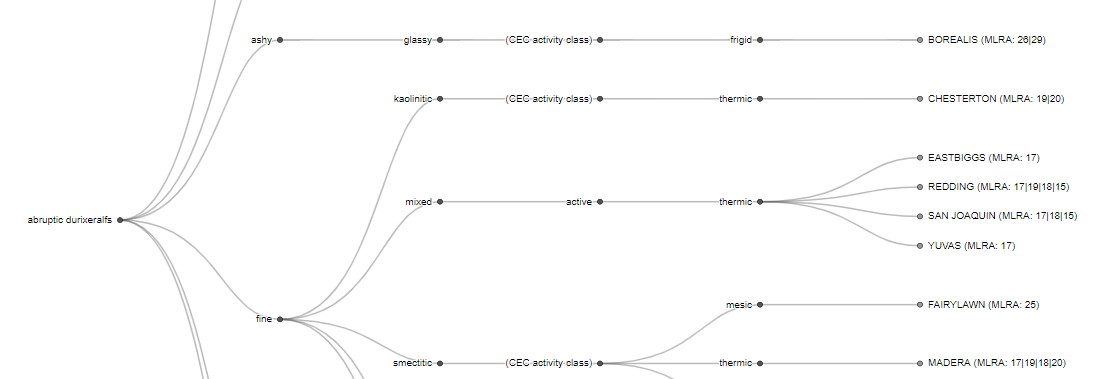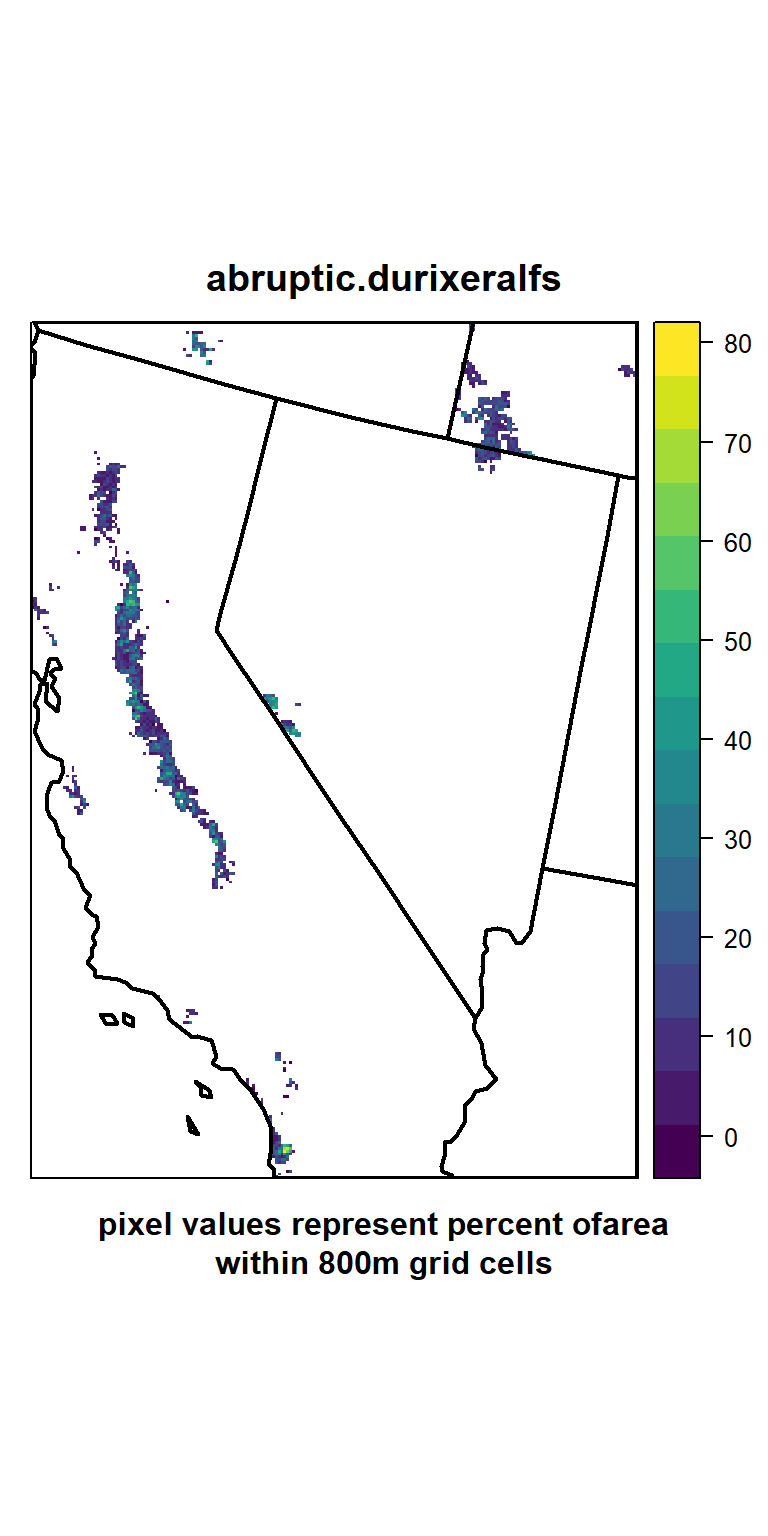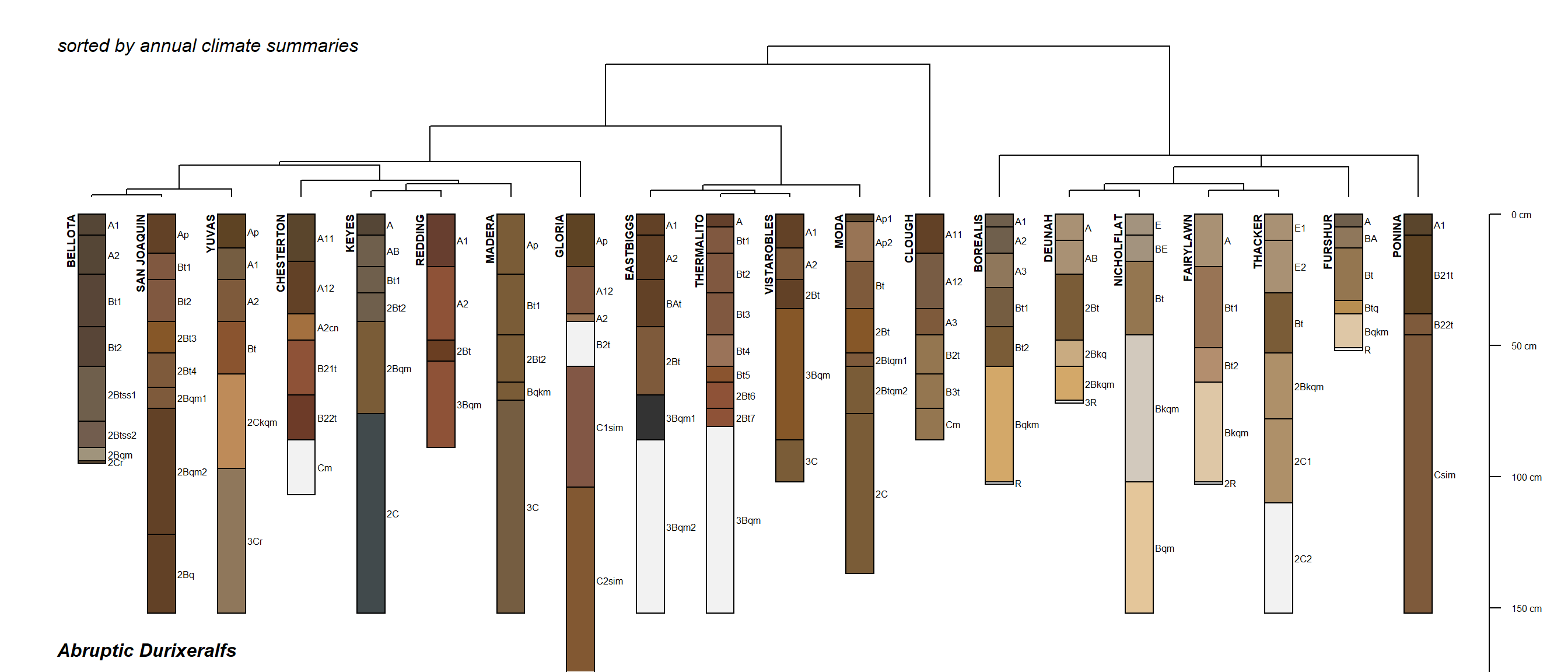# 1 Introduction

Soil Taxonomy is a theoretical framework for organizing soil information, typically at scales coarser than most land management decisions. Soil series are a practical (but limited in geographic scope) framework for organizing soil/landscape combinations close to the scale of land management decisions.

So, what is the practical manifestation of a single subgroup from Soil Taxonomy (abruptic durixeralfs), in terms of soil series concepts?

The following code, links, and notes may serve as a starting point for those interested in learning more about Soil Taxonomy. This is a work in progress.

``````# AQP suite, be sure to use the development versions from GitHub
library(aqp)
library(soilDB)
library(sharpshootR)

# used for visualization
library(latticeExtra)
library(tactile)
library(cluster)
library(ape)
library(FactoMineR)

# mapping a subgroup
library(sp)
library(sf)
library(spData)
library(rasterVis)
library(viridis)

# subgroup "explainer"
library(SoilTaxonomy)``````

# 2 Examples

Get a snapshot of the Soil Classification database. This contains the current classification for all soil series.

``````# latest version, synced quarterly
u <- 'https://github.com/ncss-tech/SoilTaxonomy/raw/master/inst/extdata/SC-database.csv.gz'
tf <- tempfile()
SC <- read.csv(gzfile(tf), stringsAsFactors = FALSE)``````

Identify all of the soil series associated with a specific subgroup taxa: abruptic durixeralfs and download basic morphology and climate summaries via `fetchOSD`.

``````# select series names for a single subgroup
s <- SC\$soilseriesname[which(SC\$tax_subgrp == 'abruptic durixeralfs')]

# get OSD morphology and extended summaries
osd <- fetchOSD(soils = s, extended = TRUE)``````

## 2.1 ST Explainer

Explain subgroup taxa via SoilTaxonomy package.

``cat(explainST('abruptic durixeralfs'))``
```abruptic durixeralfs
|        |   |  |
abrupt textural change
|   |  |
presence of a duripan
|  |
xeric SMR
|
soils with an argillic, kandic, or natric horizon
```

## 2.2 SoilWeb seriesTree ApplicationLink to SoilWeb subgroup taxa tree for abruptic durixeralfs.

## 2.3 Taxa Extent Maps

``````# get 800m extent map
e <- taxaExtent('abruptic durixeralfs', level = 'subgroup')

# aggregate via focal mean using 5x5 moving window
# visualization purposes only
a <- aggregate(e, fact = 5)

data("us_states")
us_states <- as(us_states, 'Spatial')
us_states <- spTransform(us_states, CRS(projection(e)))
us_states <- crop(us_states, e)

# simple figure
levelplot(
a,
margin = FALSE,
scales = list(draw = FALSE),
col.regions = viridis,
main = names(a),
sub = 'pixel values represent percent ofarea\nwithin 800m grid cells',
panel=function(...) {
panel.levelplot(...)
sp.polygons(us_states, col='black', lwd=2)
}
)``````## 2.4 Data via soilDB::fetchOSD

Cluster series based on annual climate summaries but don’t plot it yet.

``````# control centers symbol and size here
res <- vizAnnualClimate(osd\$climate.annual, s='SAN JOAQUIN', IQR.cex = 1.1, cex=1.1, pch=18)``````

Series associated with abruptic durixeralfs subgroup, arranged according to annual climate summaries.

``````par(mar=c(0,0,1,1))
plotProfileDendrogram(osd\$SPC, clust = res\$clust, scaling.factor = 0.075, width = 0.2, y.offset = 0.5)
mtext('Abruptic Durixeralfs', side = 1, at = 0.5, adj = 0, line = -1.5, font=4)
mtext('sorted by annual climate summaries', side = 3, at = 0.5, adj = 0, line = -1.5, font=3)``````### 2.4.1 Annual climate summaries

``````# display annual climate summary
trellis.par.set(plot.line=list(col='RoyalBlue'))
print(update(res\$fig, layout=c(4,2), sub='Abruptic Durixeralfs'))``````